# Postorder Traversal of binary trees

To postorder a non-empty binary tree, below operations are preformed recursively of each node

1. traversing the left subtree
2. traversing the right subtree
3. visiting the root nodethe word “post” specifies that the root node is accessed last to any other node from the left and right subtrees

let’s see one example: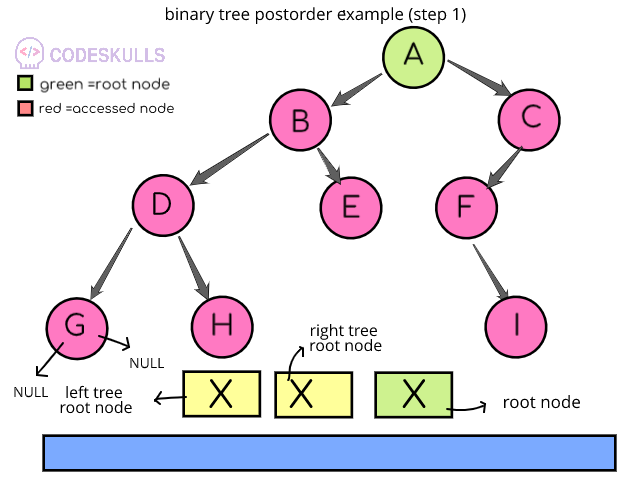step 1: currently search pointer is pointed to the node A, the root node is now node A, the first priority is the left subtree, so search pointer moves to node B, but node B has left subtree, search pointer goes to the leaf node G because there are no sub-nodes to node G, now this node G will be accessed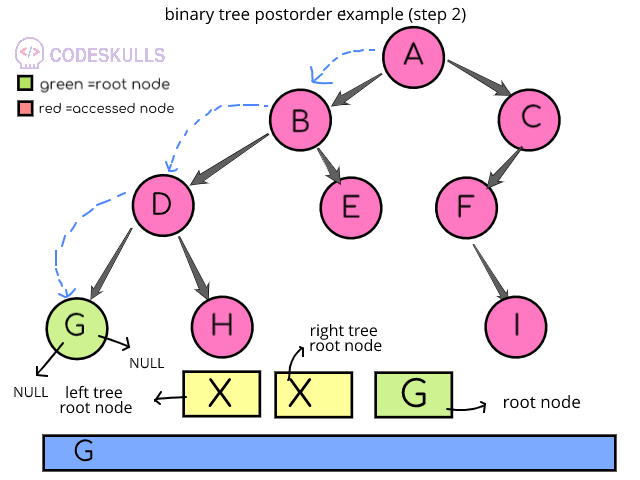step 2: search pointer goes to the leaf node G, because there are no sub-nodes to node G, now this node G will be accessed, search pointers goes back to parent node Dstep 3:search pointer is shown at node D, node D is the root node, the postorder operation is applied on the left subtree of node D , next the priority goes t the right subtree H and accessed it , H doesn’t have any sub-nodes, search pointer goes back to parent node D and root node D will be accessed at the last according to the priority order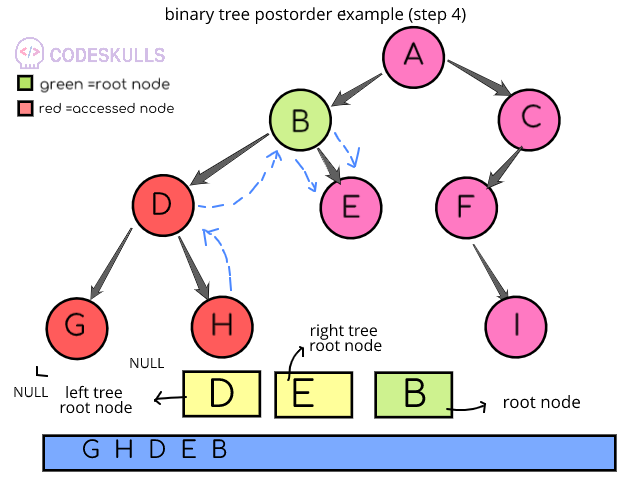step 4:search pointer will be moved from node D to the node B because node B is the parent node of node D and grandparent node of node H , now the root is at node B , post order operation is done on the left subtree of the node B, next priority is right subtree, node B’s right subtree contains single node E, this node E will be accessed, node E is doesn’t have any sub-trees so search pointer moves back to node B and access it because postorder operations are completed on both subtrees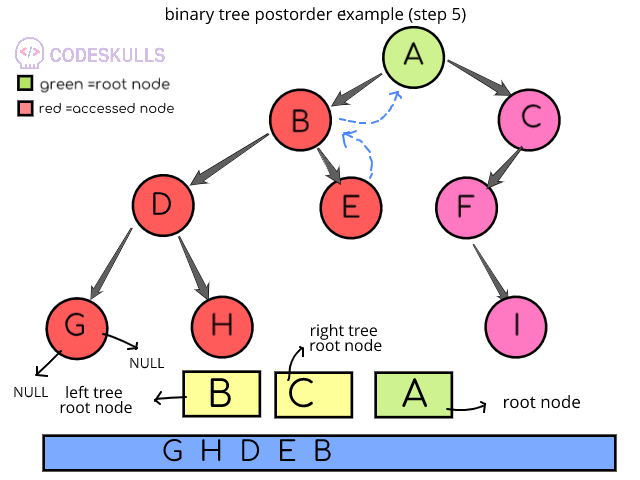step 5:search pointer is at node A, the postorder operation is completed for left subtree B and according to priority next will be right subtree (node C)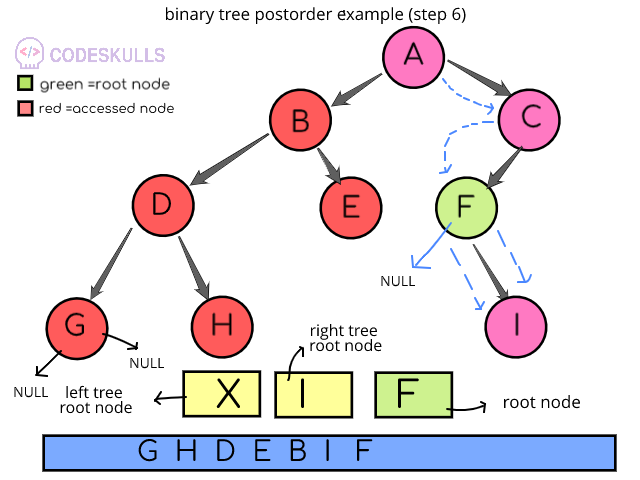step 6:serach pointer moves from node A to C , and at node C there is a left sub-tree F, search pointer movies to node F, now node F is the root node, there is no left subtree for node F , next priority goes to right subtree node I and node I will be accessed, node I doesn’t have any subnodes so search pointer moves to the parent node F , after; left and right subtrees next priority goes to root node F, so node F will be accessedstep 7:search pointer goest to the node C because this the parent node for node F, now root node is node C, the postorder operation is completed for the left subtree F, then next priority is right subtree but there is no right subtree, so next will be root node C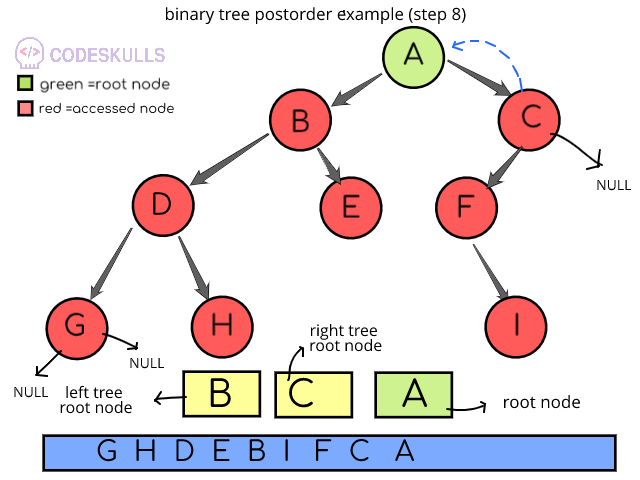step 8: pointer goes to the node A because this the parent node for node C, now root node is node A, post order operation is complete for both left and right subtrees of the node A, next priority will be itself root node A, so now root node A will be accessed

Last updated on by vishal devxo